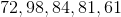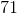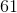## Example Questions

← Previous 1

### Example Question #1 : How To Find Median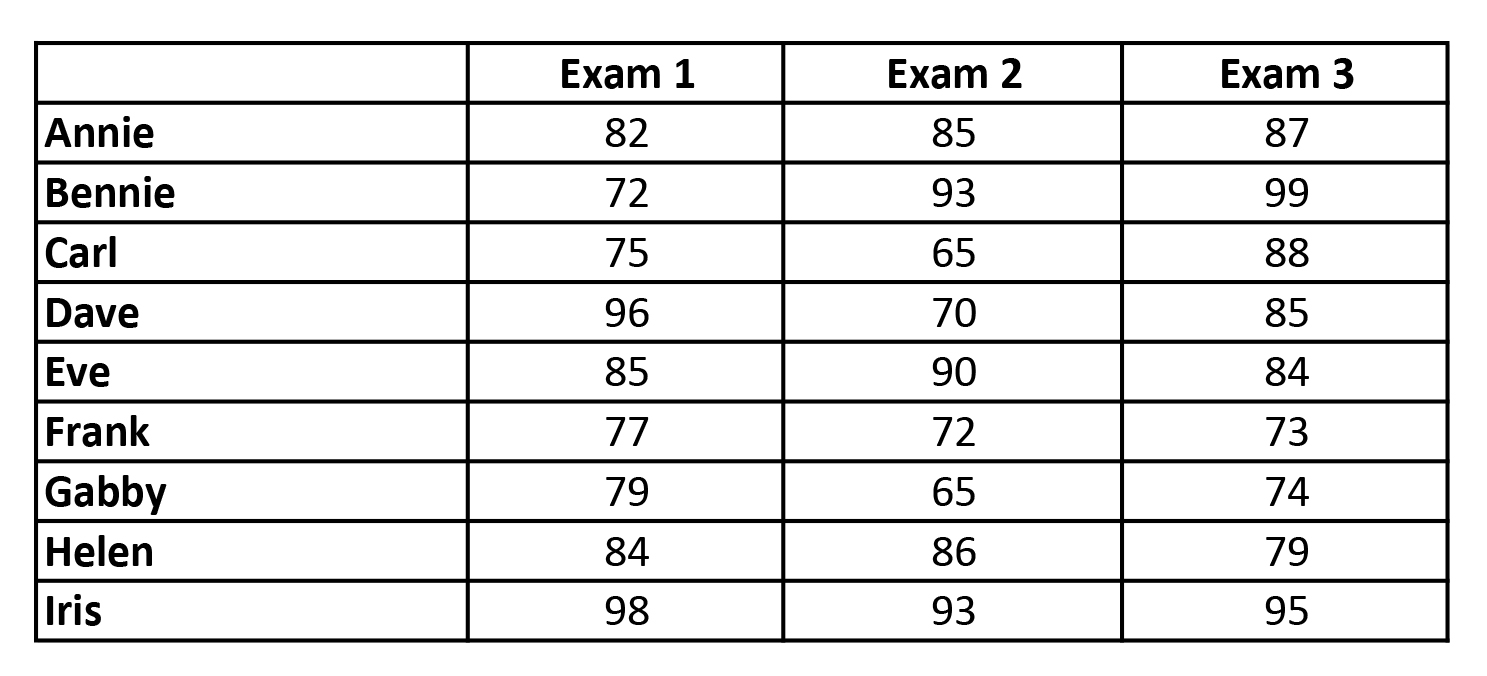Refer to the chart above. What is the Median of Exam 1?

84

85

83

79

82

82

Explanation:

To find the median, one must arrange all the heights from the lowest to the highest value and then pick the middle value.

All Values: 82 72 75 96 85 77 79 84 98

In Order: 72 75 77 79 82 84 85 96 98

Median: 82

### Example Question #2 : How To Find Median

Find the median of the given set of data:

{1,4,3,2,5,6,6}

4

3

6

1

2

4

Explanation:

First you must order the elements from least to greatest. In order, the set is {1,2,3,4,5,6,6}. There are 7 terms in this set, so the median will be the 4th term of the set, which happens to be 4.

### Example Question #3 : How To Find Median

Find the median of this set of data:

{1, 2, 3, 4, 5, 6}

4

2

4.5

3.5

3

3.5

Explanation:

When given an odd number of terms in a set, the middle term is the median.

However, if the number of terms is even, there are 2 middle terms, so the median is the average of the 2 middle terms. (3 + 4)/2 = 3.5

### Example Question #1 : How To Find Median

43, 83, 54, 35, 77,,If the median of the set of numbers is 54 and the mean is 56, which of the following could be the values ofand?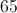and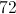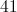and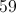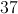and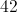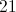and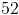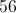and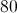andExplanation:

The median is the numerical value separating the higher half of the data sample from the lower half. Rearrange the data set by increasing value: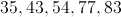In order for 54 to remain the median, either:

1. One of the variables must be greater than 54 and the other must be less than 54.

or

2. Both variables muat be equal to 54.

This eliminates all answer choices except 41 and 59. The mean of the data set including these two values is 56.

### Example Question #1 : How To Find Median

The following are the points scored on a test by students in a class:

5,9,11,11,12,13,13,13,18,20

Which of the following numbers is the mode?

13

14

12

15

11

13

Explanation:

13 is the correct answer because it occurs three times and occurs more than any other grade.

### Example Question #6 : How To Find Median

Find the median of the following set of numbers: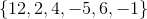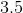Explanation:

Find the median of the following set of numbers:The median is the middle number in a group of numbers listed in order. To find the median, start by listing the numbers in increasing order: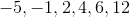Then find the middle number. In this case, there are two "middle numbers,"and, so the next step is to average them.

Therefore, the median is.

### Example Question #7 : How To Find Median

67, 73, 85, 83, 80, 73, 94, 65, 80, 73, 98, 59, 76

The list above shows a ninth grader's grades for the academic year. What is the difference between the median and the mode of these grades?Explanation:

To find the median, sort the numbers from smallest to largest:

59, 65, 67, 73, 73, 73, 76, 80, 80, 83, 85, 94, 98

The median is the middle value in a list of numbers, it is the number separating the higher half of a data sample or a list of numbers from the lower half.

The median of the grades is 76.

The mode is the value occurring most often. The most occurring value in the list of numbers given is 73. So, the mode is 73.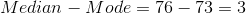### Example Question #8 : How To Find Median

In a row ofstudents, each student receives a number of chocolates equal his or her position in the line, multiplied by.  What is the median amount of chocolates received by this group?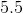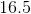There is no median.Explanation:

To begin, think through the scenario of your line, it is made up of ten students as thus: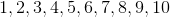Since there is an even number of students, your median will be the average of the amounts given to studentsand.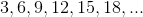Thus, you know that the median must be: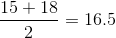### Example Question #9 : How To Find Median

Four items are purchased from an electronics store. The first item costs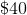and each successive item costs twice that of the previous one. What is the median cost of these four items?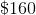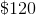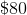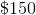There is no medianExplanation:

To start, you should list out the prices of your items: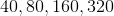Now, recall that the median of a group of numbers is the middle value. For four numbers, there is (strictly speaking) no middle. Therefore, you need to add together the values in the second and third place and find their average. This is: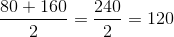### Example Question #10 : How To Find Median

What is the median of the following list oftest scores: